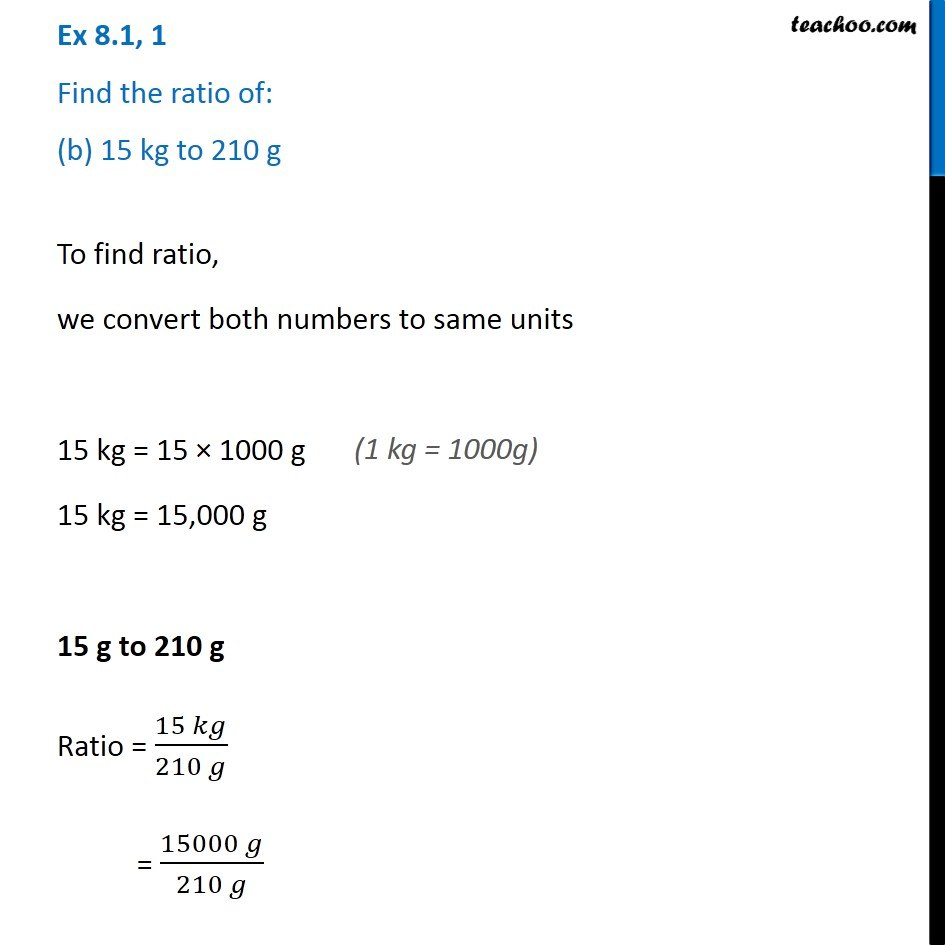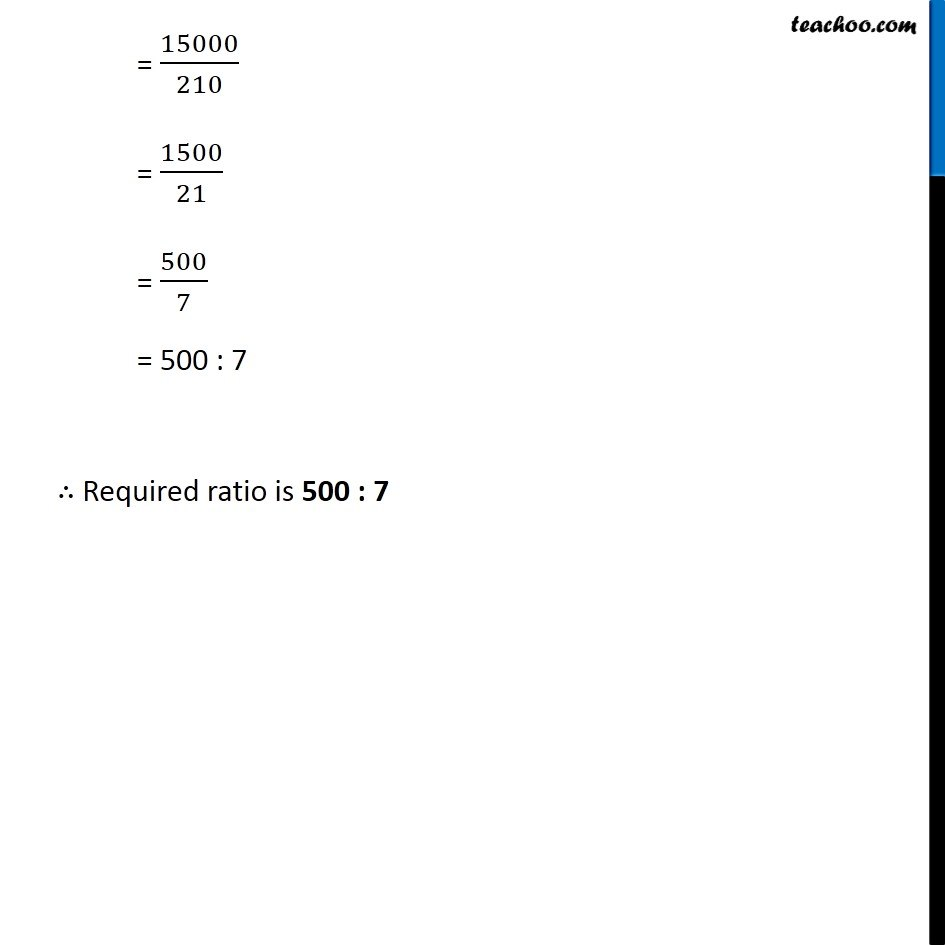Finding Ratios

Chapter 7 Class 7 Comparing Quantities
Serial order wiseLearn in your speed, with individual attention - Teachoo Maths 1-on-1 Class

### Transcript

Question 1 Find the ratio of: (b) 15 kg to 210 g To find ratio, we convert both numbers to same units 15 kg = 15 × 1000 g 15 kg = 15,000 g 15 g to 210 g Ratio = (15 𝑘𝑔)/(210 𝑔) = (15000 𝑔)/(210 𝑔) = 15000/210 = 1500/21 = 500/7 = 500 : 7 ∴ Required ratio is 500 : 7